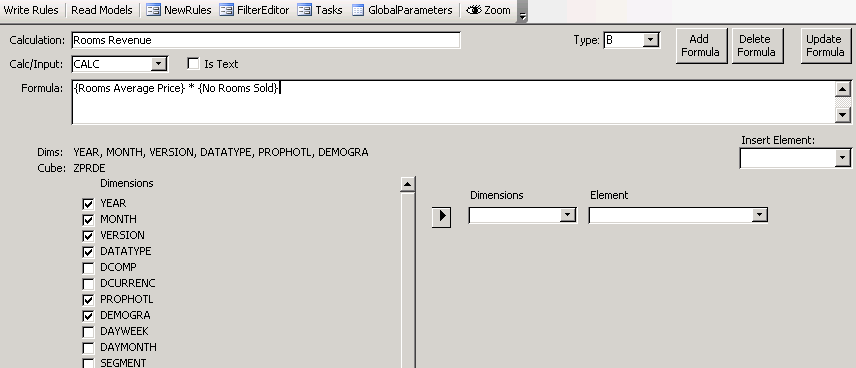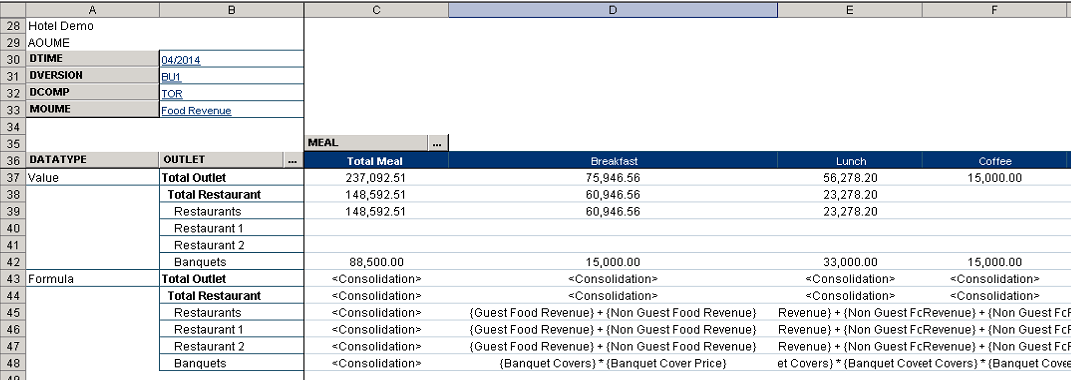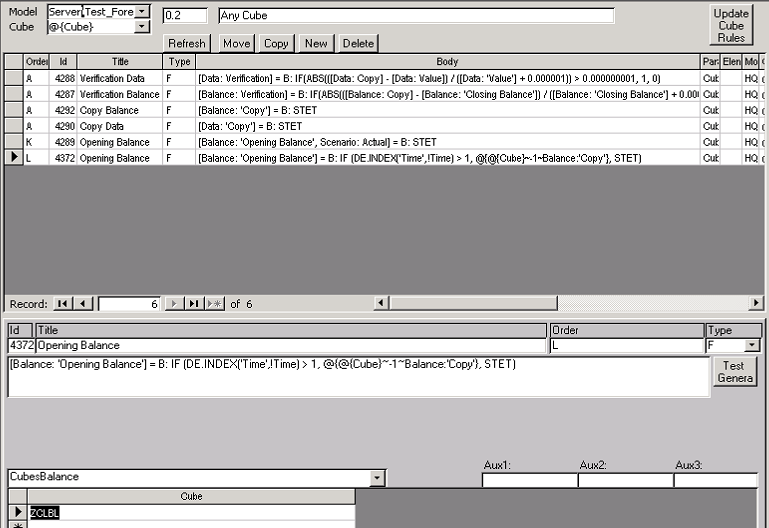# Building Complex Financial Models for Infor Olap Using CIPHER’s Calculation Editor

Why do we need a more advanced formula paradigm for Infor OLAP?

As powerful as the rules in Infor OLAP are, it is not easy to build a complex system using just the rule editor and the syntax it provides. As the number and complexity of rules grow it quickly becomes difficult to manage. Rules become hard to understand and maintain since they are scattered among different cubes. Making dimension changes in a cube requires a lot of rule rewriting.

## There is a better way

CIPHER’s Calculation Editor is not simply a new rule editor; in fact it is a paradigm change. We introduce what we call Calculations.

### Rules vs Calculations

In Infor OLAP rules belong to a cube. Each cube has a set of rules, and each rule calculates a certain slice of the cube. Usually, but not always, there is a dimension in each cube that represents the measures of that cube like Sales, Volume, Price.Calculations are measures but they are not associated to a specific cube, they belong to the whole model. Calculations are model-wide measures as opposed to cube-wide. Calculations have formulas that operate on other Calculations in the model. The Calculation Editor knows the dimensionality of each Calculation and places them in the corresponding cubes. In fact it creates and removes the cubes as needed. Calculation’s formulas are translated into rules, always using the correct references.The user defines the dimensionality of each Calculation by selecting the appropriate dimensions. Changing the dimensionality is just a matter of clicking a checkbox.The paradigm change is that, instead of creating cubes and writing rules for each individual cube following a rigid design, the developer creates Calculations, enters the formulas and then decides which dimensions are appropriate for each Calculation. The developer can then test and adjust the model changing the Calculations’ dimensionality and formulas. With a click of a button the Calculation Editor updates the model changing the cubes and placing the Calculations and formulas in the right cubes.

This paradigm change means that developing a financial model can now be more of an iterative process where a prototype evolves into the solution required. A process where there are no big penalties for making structural changes to the original design. The resulting methodology is more in tune with the realities of creating financial models.

The new Calculation Editor also improves in the readability and maintainability of the formulas.

## Infor Rules can be hard to understand.

### Basic Syntax.

In their basic form, Infor rules can be easy to write and easy to understand, for instance:

`    [Sales] = [Volume] * [Price]`

This way of referencing cells within the same cube (internal references) is very easy to read and code. The rule parser even makes an effort to simplify the syntax (syntactic sugar) by determining the dimension based on the element name, whenever possible. Without the syntactic sugar the same rule would look like this:

`    ['Measure':'Sales'] = ['Measure':'Volume'] * ['Measure':'Price']`

Not as readable as the first example but this one has the advantage of not being ambiguous. The first version is easier to read but it risks failing in the future if one of the referenced elements becomes ambiguous which can happen if an element with the same name is added to another dimension.

### Let’s compare to CIPHER’s Calculation Editor.

CIPHER’s Calculation Editor uses a preprocesor that converts from Calculation syntax to Rule syntax. The above example looks like this:

`    {Sales} = {Volume} * {Price}`

That’s it, that simple. No need for syntactic sugar. And no risk of future ambiguity.

### DB Calls.

So far so good, but syntax gets a bit more complicated when DB calls are involved (external references).

Typically DB calls are used when information from another cube needs to be fetched. For instance in the case above the price could reside in the cube PRICES:

`    [Sales] = [Volume] * DB('PRICES', !Year, !Month, !Version, !Product, !Region, 'Price')`

Suddenly the syntax is not as elegant, all the punctuations and dimension references make it harder to read. There is no syntactic sugar anymore, all commas, quotes, parentheses and exclamation points are unavoidable.

### Compare to CIPHER’s Calculation Editor.

In Calculation syntax the above example looks like this:

`    {Sales} = {Volume} * {Price}`

Yes. External references look just like internal references.

### Nested Calls.

In some cases DB calls need to be nested, making it even harder to understand. For instance:

```    [Sales] = [Volume] *
DB('PRICES', !Year, !Month, !Version, !Product
, DB('REGIONS', !Year, !Version, !Customer, 'Region')
, 'Price'
)
```

Things can get hairy pretty quickly.

### Compare to CIPHER’s Calculation Editor.

`{Sales} = {Volume} * {Price; Region:{Region} }`

And we have only scratched the surface here, in real models rules get a lot more complicated. This is what an actual rule from Infor Consolidation looks like:

```
[DLCURR:'USD',DDATA:'Balance Sheet']=
IF(DB('TCOMPPA',!DYEAR,!DPERIOD,!DVERSIO,!DCOMPC,'Currency')@='',0,
IF(DB('TCOMPPA',!DYEAR,!DPERIOD,!DVERSIO,!DCOMPC,'Currency')@=!DLCURR,
[DLCURR:'LC',DDATA:'Balance Sheet'],
IF(GETATTR('DACOUNT',!DACOUNT,1,INSRT(DB('TCONSPA',!DYEAR,!DPERIOD,!DVERSIO,'global',!DCOMPC,'global','currency conversion method'),'Method', 7))@='',
[DLCURR:'LC (scaled)',DDATA:'Balance Sheet'],
IF(GETATTR('DACOUNT',!DACOUNT,1,INSRT(DB('TCONSPA',!DYEAR,!DPERIOD,!DVERSIO,'global',!DCOMPC,'global','currency conversion method'),'Method', 7))@='WA',
[DDATA:'Historical Translation Value'],
[DLCURR:'LC',DDATA:'Balance Sheet']
/ IF(SUBST(GETATTR('DACOUNT',!DACOUNT,1,INSRT(DB('TCONSPA',!DYEAR,!DPERIOD,!DVERSIO,'global',!DCOMPC,'global','currency conversion method '),'Method',7)),1,1)@<>'H',
DB('TRATES',!DYEAR,!DPERIOD,!DVERSIO,!DLCURR, GETATTR('DACOUNT',!DACOUNT,1, INSRT(DB('TCONSPA',!DYEAR,!DPERIOD,!DVERSIO,'global',!DCOMPC,'global','currency conversion method'), 'Method', 7)), DB('TCOMPPA',!DYEAR,!DPERIOD,!DVERSIO,!DCOMPC,'Currency')),
IF(SCAN('HI',GETATTR('DACOUNT',!DACOUNT,1,INSRT(DB('TCONSPA',!DYEAR,!DPERIOD,!DVERSIO, 'global',!DCOMPC,'global','currency conversion method'),'Method',7)))>0,
[DCLEVEL:'HB I',DDATA:'Historical Exchange Rate'],
[DCLEVEL:'HB I',DDATA:'Historical Exchange Rate',DINTCO:'External']))
* DB('TRATES',!DYEAR,!DPERIOD,!DVERSIO,!DLCURR,'units',DB('TCOMPPA',!DYEAR,!DPERIOD,!DVERSIO,!DCOMPC,'Currency'))
))))
```

Yikes! There are 11 DB calls in that one rule alone! Make sure to check how long some of those lines are.

### Compare to CIPHER’s Calculation Editor.

There are no DB calls

```{Balance Sheet; DLCURR: 'USD'} =
IF({Currency} @= '', 0,
IF({Currency} @= !DLCURR,
{Balance Sheet; DLCURR: 'LC'},
IF(GETATTR('DACOUNT',!DACOUNT,1,INSRT({Currency conversion method},'Method', 7))@='',
{Balance Sheet; DLCURR: 'LC'},
IF(GETATTR('DACOUNT',!DACOUNT,1,INSRT({Currency conversion method},'Method', 7))@='WA',
{Historical Translation Value},
{Balance Sheet; DLCURR: 'LC'}
/ IF(SUBST(GETATTR('DACOUNT',!DACOUNT,1,INSRT({Currency conversion method},'Method',7)),1,1)@<>'H',
{Rate; DEXRATE: GETATTR('DACOUNT',!DACOUNT,1,INSRT({Currency conversion method},'Method', 7)); DCCURR: {Currency}},
IF(SCAN('HI',GETATTR('DACOUNT',!DACOUNT,1,INSRT({Currency conversion method},'Method',7)))>0,
{Historical Exchange Rate; DCLEVEL:'HB I'},
{Historical Exchange Rate; DCLEVEL:'HB I'; DINTCO:'External'})
* {Rate; DEXRATE: 'units'; DCCURR: {Currency}}
))))```

### Code Repetition

In the currency conversion rule above you can notice another issue, and that is code repetition. The rule language does not allow for intermediate variables and does not implement expansion macros. What that means is that every value required needs to be repeated every time it is used. How many times can you find the following call repeated above?

`GETATTR('DACOUNT',!DACOUNT,1,INSRT(DB('TCONSPA',!DYEAR,!DPERIOD,!DVERSIO,'global',!DCOMPC,'global','currency conversion method'),'Method', 7))`

5 times in just this particular case! And it is not the only part that repeats over and over. Most of that rule is a repetition of some sort. To make matters worse there are many rules that share the same bits of code among them. This makes the rules very hard to read and understand.

### Compare to CIPHER’s Calculation Editor.

The Calculation Editor has an expansion macro feature that allows for code reusability.

```ConversionMethod = GETATTR('DACOUNT',!DACOUNT,1,INSRT({currency conversion method},'Method', 7))

{Balance Sheet; DLCURR: 'USD'} =
IF({Currency} @= '', 0,
IF({Currency} @= !DLCURR,
{Balance Sheet; DLCURR: 'LC'},
IF(@{ConversionMethod} @='',
{Balance Sheet; DLCURR: 'LC'},
IF(@{ConversionMethod} @='WA',
{Historical Translation Value},
{Balance Sheet; DLCURR: 'LC'}
/ IF(SUBST(@{ConversionMethod}, 1,1)@<>'H',
{Rate; DEXRATE: @{ConversionMethod}; DCCURR: {Currency}},
IF(SCAN('HI',@{ConversionMethod})>0,
{Historical Exchange Rate; DCLEVEL:'HB I'},
{Historical Exchange Rate; DCLEVEL:'HB I'; DINTCO:'External'})
* {Rate; DEXRATE: 'units'; DCCURR: {Currency}}
))))```

Much more concise. Macro definitions are global so they can be reused in many rules. They also allow passing parameters and some basic logic.

### Time Shifting

Another case when DB calls are needed is when one of the referenced elements is not constant but calculated. For instance if we wanted to increase the price from the prior year by 5% the formula for 2010 could look like this:

`[Price, 2010] = 1.05 * `

Simple enough but not generic. A generic formula that works for any year would be:

```    [Price] = 1.05 *
DB('PRICES', STR(NUMBR(!Year) - 1, 4, 0)
, !Month, !Version, !Product, !Region, 'Price')```

Notice the formula for prior year.

Going back one year is not so bad. But what if you need to go back 3 months instead? We would need to check when the year changes. An ad hoc rule would look like this:

```    [Price] = 1.05 *
DB('PRICES', IF(NUMBR(!Month) > 3, !Year, STR(NUMBR(!Year) - 1, 4, 0))
, STR(MOD(NUMBR(!Month) - 3 + 11, 12) + 1), 2, 0)
, !Version, !Product, !Region, 'Price')```

Again, not generic enough. What if we wanted to go back (or forward) by a variable number of months? And how about if going back takes us beyond the start of the forecast and we needed to fetch from the Actual Scenario instead? No need to show the actual rule, you get the idea, reality is more complicated than that.

### Compare to CIPHER’s Calculation Editor.

The above example looks like this:

`    {Price} = 1.05 * {Price;-3}`

Notice how easy it is to go back and forth in time. It can even use a variable or calculated number of periods and it can handle fetching the actual scenario when needed.

## Rule Maintenance

### Cube and Dimension structure

Readability in rules is important but there is a much bigger issue with the current rule syntax.

DB calls require that each dimension be specified in the same order as the cube is defined. That means that if dimensions are added, removed or the order of the dimensions changes, rules would cease to work.

In the example above if we wanted to remove the REGION dimension from the price cube, all rules that reference that cube would stop working. In a large model that could mean going through tens of rules in different cubes adjusting for the change.

Because of this, when modeling with Infor OLAP the developer needs to determine very early on the correct structure of cubes and the dimensionality of each measure. Failing to do so will result in lots of hours rewriting rules.

It is not so easy to predefine the cubes and dimensions of a complex financial model. During the development of a financial model it is not uncommon to make changes to the dimensionality of the cubes. In fact it is a given. Sometimes during testing the users may discover that they need more granularity and so a dimension is added. Or they may decide to split a dimension into two or more. If the model has performance issues it could be wise to remove some dimensions.

Even after a model has gone into production it may require changes. Financial models are not static, they evolve with the company and with the requirements of the users and management.

### Compare to CIPHER’s Calculation Editor.

CIPHER Calculation Editor knows the structure of every cube and writes the DB calls correctly every time. Changing dimensions in a cube does not require rewriting the rules. Furthermore, not even moving Calculations from one cube to another requires any recoding. It is all done by the Calculation Editor

## Other Unique Features

### Formula Discovery

End users working with a financial model, specially a newly developed one or one they are not familiar with, can be mystified about the numbers produced by the system. Good documentation of course helps. Since Calculation Editor formulas reside in a relational table, documenting the financial model is really easy. Formulas can easily be sent to the printer or used as a source for an online documentation system.

But Calculations have another ace up the sleeve and that is formula discovery. Formula discovery actually lets the user see the formula used in each cell directly from the OLAP model. Users of Office Plus or Application Studio can immediately retrieve the actual formula that calculated the values they are seeing or they can see whether the number is a <Consolidation> or an <Input>.### Freeze/Unfreeze functionality

Infor OLAP is extremely fast and efficient for aggregating data, efficiently adding up figures through the hierarchies to provide totals. Other types of calculations are not as fast and they are affected by the number and size of dimensions. Also whenever any piece of data changes, Infor OLAP for good measure starts over from scratch which means it does not save already calculated values even if those are not going to change. This can make financial models too slow to meet expected response times.

CIPHER’s Calculation Editor implements freeze functionality that allows the user to save the already calculated results as input data. This prevents the OLAP engine from having to calculate from the beginning every time. The user can select which slice of the data to freeze: it can be up to a certain period, a specific version or property or up to a certain section in the model. For instance if the revenue section for company C has already been finished that section can be frozen and the user can continue working in the budget for the expenses section enjoying a much faster response time.

By the end of the budgeting cycle the whole model can be frozen which will make the reports as fast as any typical Infor OLAP report based on only aggregating data.

Many times financial models can be too rigid in their calculations to allow for real life requirements. In those cases it is good to have the possibility to adjust or override the values calculated by the model. Normally in Infor OLAP that is not possible unless a change is made in the specific rule(s). Requirements like these are hard to foresee and they usually arise during the heat of the budgeting cycle when pressure is up and there is little time to be doing changes to the model logic.

For those situations the Calculation Editor provides the user with the capability of making adjustments to any of the calculated values. Every Calculation formula can provide this functionality without having to change or clutter the formula in any way.

### Actual and Forecast merging

CIPHER’s Calculation Editor allows the user to set the starting period for each budget/forecast scenario. That allows it to automatically copy data from the Actual scenario up to the start of the budget and then calculate the subsequent periods using the formulae. That way the user only needs to load actual figures in one scenario and have multiple forecasts each starting in a different date that show a combination of actual and forecast data. All reports will show this actual/forecast combination.

### General Ledger Assembly

Having used the powerful formulas to do the Calculations, the last step is to assemble the General Ledger taking the values from the different Calculations and allocating them into the proper account and department codes. This allows for P&L, Balance Sheet and Cash Flow reporting using the same structure as the actual data, which makes comparing Actual vs Budget/Forecast a lot easier. This mechanism also allows the Calculations and formulas to use natural names instead of numerical Account codes for greater clarity.

During the G/L Assembly the user can choose calculations, apply ratios/multipliers, input data, and even use other accounts to allocate the values.

### Validations

Calculations come with a predefined slot for validations. The model can therefore validate for data completeness, consistency and compliance. For instance, a formula can verify that every product with volume different than zero has a corresponding price and a unit cost entered. Validations roll up to the top of the hierarchies to make it easy to spot the issues by looking at the big total, so if a validation flag is on, the user can drill down to locate the exact point where the issue is.

### Good old Rules, but global

CIPHER’s Calculation Editor also allows the user to write Infor OLAP rules, not just Calculations. Rules can be necessary when a formula does not apply to a specific Calculation but is oriented to several of them. In fact these types of rules are used extensively to provide much of the functionality mentioned above. These rules use the macro expansion functionality which means that they are much more powerful than Infor rules. For instance:

A rule can be global and apply to all cubes or only to cubes that meet a certain condition.

• Do not have to use the rigid DB calls.
• Can use logic to select which dimension elements to apply to.
• Can use any relational source to create special rules.